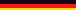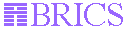Fast dieselbe Seite auf Deutsch

# Bit-Vector Research PageThis page contains actual information about developments and results concerning the theory of bit-vectors with a special focus to the construction of solver algorithms.

 General Information

Bit-vectors are - in the context of hardware verification - data structures, which represent elements of a mutisorted logic (i.e. a bit-vector can be of the type bvec, bvec,...). The width 0 is illegal.The bits are counted from left to right, starting with 0. In contrast to arrays, bit-vectors are considered finite objects, each position containing a value out of a binary alphabet.
Typical operations are

• Extraction :: <bvec> [<nat>:<nat>]
e.g. x[0:6] means the seven leftmost bits of x (x: bvec[n], n>= 7)
• Concatenation :: <bvec> o <bvec>
t.i. the associative concatenation of bit-vectors (like x o y o z)
• Fill :: Fill[<nat>](<Bit>)
Denoting a bit-vector of the width n, containing the same bit at each position. This bit can be a constant or a variable.
Additionally, the following operations are found frequently:
• Boolean operations :: NOT <bvec>, <bvec> AND <bvec> , ...
are of course only valid, if the arguments have the same width
• arithmetical operations :: + *
Usually, an arithmetic modulo 2^n is intended, where n is the width of the arguments. The leftmost bit is considered to be the least significant one.
• Bitextract :: <bvec> (<nat>)
Produces - as expected- a bit
• bv2nat(<bvec>) and nat2bv[<nat>](<nat>)
Transformation of bit-vectors to natural numbers (and vice versa);
The function nat2bv gets usually the width of the resulting bit-vector as an argument
The main focus of the research in Ulm is to construct efficient solvers for a rich theory of bit-vector equations.
As it turnes out, it is a crucial point whether to allow variable width (e.g. vie introducing a dependend type bvec[n], where n is a integer variable itself) or variable extraction (terms x[i:j] where i and j are integer variables). If one of these operations is permitted, the theory is no longer convex in the sense that a solution is always a conjunction of equations.

 Group members

 Complexity Results

(dvi files)

 Canonizer and Solver

Those should be build according to the requirements from the theory of congruence closure (Nelson-Oppen), explicitly formalised in the CADE-paper 1996 of Patt Lincoln, David Cyrluk und Natarjan Shankar).
A solver for simple bit-vector terms (fix extraction, known length, no boolean connectors) was given in the technical report of Harald Ruess/Oliver Möller (both Ulm) and David Cyrluk (SRI), called "An Efficient Decision Procedure for a Theory of Fixed-Sized Bit-vectors with concatenation and extraction":

The next step was the introduction of boolean operators (David Cyrluk/Oliver Möller, SRI, October 96), using ordered binary decision diagrams (for a demonstration see OBDDs ).
The algorithm (in Common Lisp) was implemented and a paper titled "An Efficient Decision Procedure for the theory of Fixed-Sized Bit-Vectors" is published in the CAV97:
And a recent result, adressing non-fixed size is found here:

You might also get a short overview on the topic by looking through the prepared

 Recent Work

In connection with the work on the diploma thesis "Solving Bit-Vector Equations - A Decision Procedure for Hardware Verification" a number of decision procedures were implemented in Allegro Common Lisp and are available here:

 gzipped tar archives, uncompress via gtar -xvzf ; to get all in one archive (73,4 KB), click here Solver: WS1S with MONA Reduced Chopper Fixed Solver Split-Chop Allows fixed width Boolean op's, Arithmetic fixed width just concatenation and extraction fixed width Boolean op's, Arithmetic [includes heuristics] variable width concatenation and (variable) extraction Size 14.6 KB 18.4 KB 22.1 KB 30.1 KB FilesContained check_via_mona.cl bvec_arith.cl bvec_structure.cl reduced_chopper.cl bvec_arith.cl bvec_structure.cl bvec_fixed_solver.cl bvec_slicing.cl bvec_bdd_solve.cl bvec_arith.cl bvec_structure.cl split_chop.cl fm_elimination.cl bvec_arith.cl bvec_slicing.cl bvec_structure.cl

The thesis itself can be obtained at Diploma Thesis. It contains a description of the solvers as well as some new complexity results. E.g. it is shown that no solver can exist for the theory of bit-vectors, if variable width, variable extraction and boolean operations are allowed.